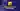In this short article, you will learn how to exit a JavaScript function.

When creating a function using JavaScript, you may want to stop its execution and exit it. To do so, these are two ways of doing it:

• by reaching the function end
• by using the `return` statement. Once your program reaches this instruction, it will exit the function by returning a value.

## Exit a JavaScript function by reaching its end

Before discovering how to exit in JavaScript, let's see when a function exits by itself.

Here is an example:

``````function writeMyName(name) {
// Print the name variable
console.log(name)
// Then, reach the end of the function
// and automatically exit it
}

writeMyName('Gaël')

// Output: "Gaël"``````

In the case above, you have a simple example. As you can see, the function only prints the `name` variable. In that case, the program exit the function after executing all the instructions.

## Return in JavaScript function

### Exit and return an undefined value

Now, let's dig a bit further and see how you can exit a function using the `return` statement. This statement allows you to leave a function once you reach it.

Let me show you an example:

``````function writeMyName(name) {
// Execute the return instruction
// and automatically exit the function
// Note: all code after a `return` isn't executed
return
// Print the name variable
console.log(name)
}

writeMyName('Gaël')

// No output``````

By default, the `return` statement send an undefined value. As an example, if you save the `writeMyName` output to a variable and print it, here what you will get:

``````function writeMyName(name) {
// Execute the return instruction
// and automatically exit the function
// Note: all code after a `return` isn't executed
return // No value = undefined
// Print the name variable
console.log(name)
}

const output = writeMyName('Gaël')

console.log(output)

// Output: undefined``````

### Exit and return a value

Now, you know how to stop a function and exit it whenever you want. But, let's imagine that you want to exit a JavaScript process and send a result value.

You can do the same as we did before, but specify a value. This value can come from a variable.

``````function writeMyName(name) {
if (name === 'Wrong') return false

// Print the name variable
console.log(name)

return true
}

console.log(writeMyName('Gaël'))

// Output:
// "Gaël"
// true

console.log(writeMyName('Wrong'))

// Output: false``````

In the example above, you're first checking the `name` variable value. If the value is "Wrong", you exit the function and send a `false` boolean value. Otherwise, you print the `name` variable, then send a `true` boolean value as a return value.

That's all about how you can exit a JavaScript function. 🚀

➡️ I help web developers improve their skills 💻 If you want to get more tips and resources about web programming -> Follow me on Twitter 🐦

Learn to code with simple and concrete examples.